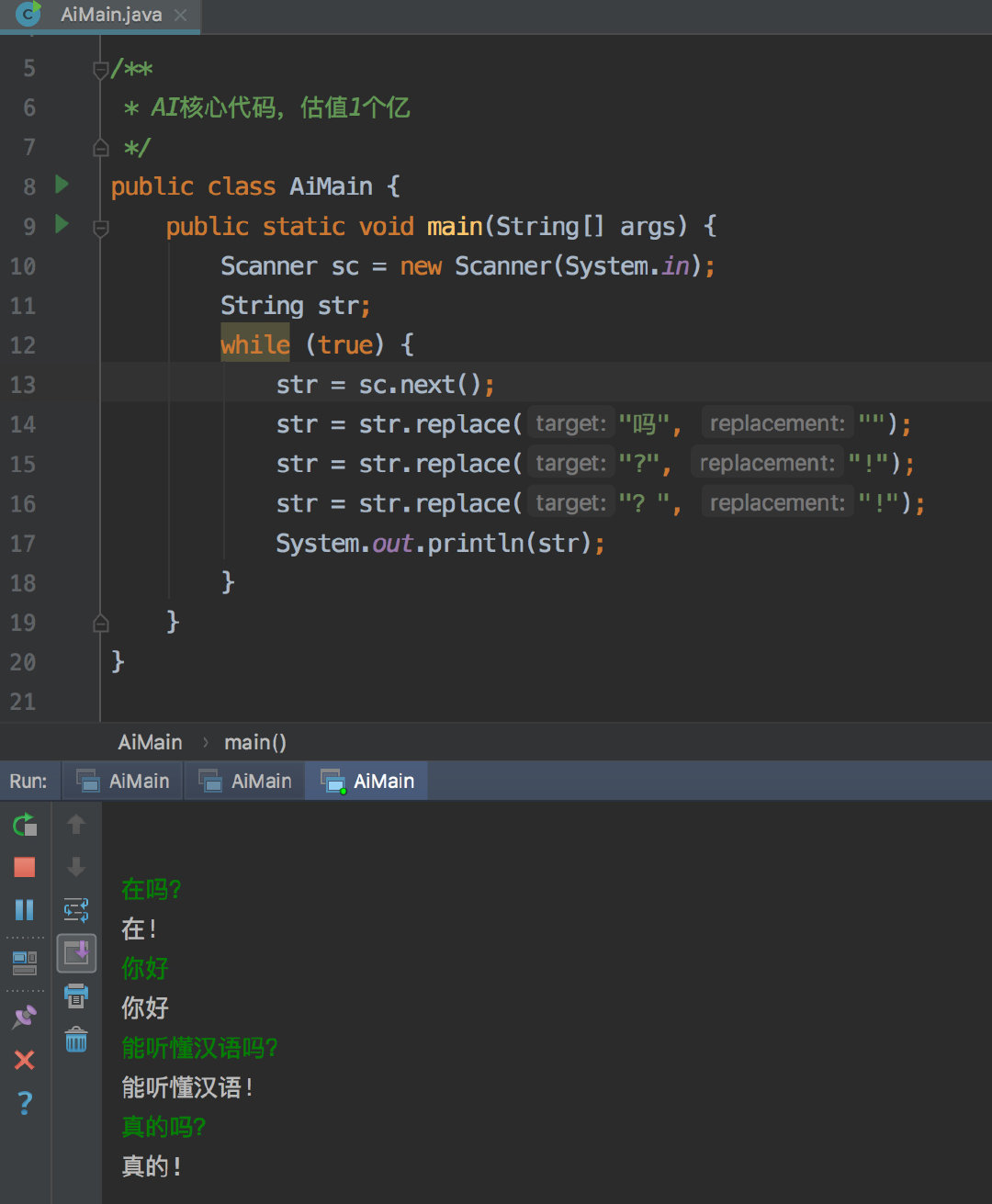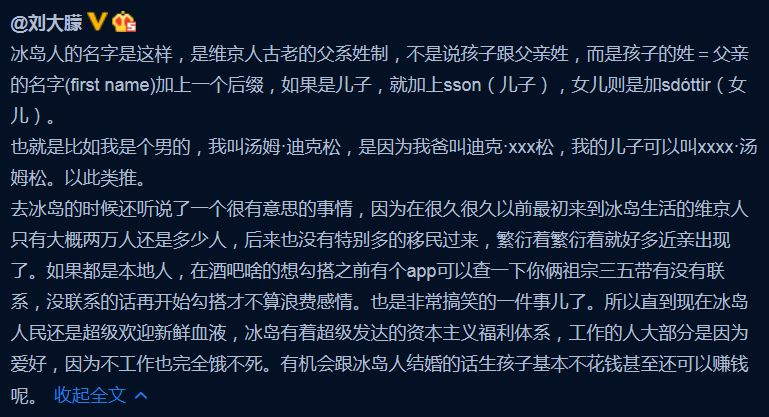# 2021年团体程序设计天梯赛赛前模拟赛4（补题）PTA 同时被 2 个专栏收录8 篇文章 0 订阅

## L1-8 估值一亿的AI核心代码 (20 分)6
Hello ?
Good to chat with you
can you speak Chinese?
Really?
Could you show me 5
What Is this prime? I,don 't know

Hello ?
AI: hello!
Good to chat with you
AI: good to chat with you
can you speak Chinese?
AI: I can speak chinese!
Really?
AI: really!
Could you show me 5
AI: I could show you 5
What Is this prime? I,don 't know
AI: what Is this prime! you,don’t know

**2.**把原文中所有独立的 can you、could you 对应地换成 I can、I could—— 这里“独立”是指被空格或标点符号分隔开的单词；
3.把原文中所有独立的 I 和 me 换成 you；

4. 对于”消除原文中多余空格：把相邻单词间的多个空格换成 1 个空格，把行首尾的空格全部删掉，把标点符号前面的空格删掉；”这个删除空格的操作，可以通过一开始预处理字符串，在普通字符串和标点符号和空格之间，都加空格来加以区分，并把它们（除了空格）分割成（利用一开始加的空格）多个字符串，最后输出字符串的时候，按照形式输出即可。第一个如果是标点，就在AI:后面先加空格。

#include<iostream>
#include<cstring>
#include<cstdio>
#include<sstream>
#include<algorithm>
using namespace std;
const int N=1010;
int main(){
int n;
scanf("%d",&n);
getchar();
while(n--){
string s,str[N],s1;
int cnt=0;
getline(cin,s);
cout<<s<<endl<<"AI:";
for(int i=0;i<s.size();i++){
if(isalnum(s[i])){       //判断字母或者数字的函数
if(s[i]!='I'){
s[i]=tolower(s[i]); //把除了'I'的大写字母都变成小写字母
}
}else{
s.insert(i," "); //把空格(标点符号)与普通字符（字母或者数字）之间加个" "， 方便下面分割成多个字符串
i++;          //因为加了个" "，字符串长度会+1，且在i后面加了个空格，不多加+1，会死循环

}
if(s[i]=='?') s[i]='!';
}
stringstream ss(s);   //这里是为了消除空格操作 ,把普通字符串与标点符号与空格根据有空格来分割
while(ss>>s1){        //这里就是消除所有空格的操作，
str[cnt++]=s1;   //把一整个字符串，根据空格分割成多个字符串
}
if(!isalnum(str)){  //如果第一个不是数字或者字母，就要提前空格与开始的AI:空格
cout<<" ";
}
for(int i=0;i<cnt;i++){
if(!isalnum(str[i])){  //这里的标点与普通字符串不分割（数字或者字母）
cout<<str[i];
}
else if(str[i]=="can"&&i+1<cnt&&str[i+1]=="you"){  //这里就是根据题意转化
cout<<" I can";
i++;        //因为是两个字符串，要再+1
}
else if(str[i]=="could"&&i+1<cnt&&str[i+1]=="you"){ //同上
cout<<" I could";
i++;
}
else if(str[i]=="I"||str[i]=="me"){  //同上
cout<<" you";
}
else cout<<" "<<str[i];  //除此之外的普通字符串，就是空格+字符串的形式输出
}
printf("\n");
}
return 0;
}


## 特立独行的幸福

​4
​​ 。

10 40

19 8
23 6
28 3
31 4
32 3

110 120

#include<iostream>
#include<cstring>
#include<algorithm>
#include<cstdio>
#include<math.h>
using namespace std;
const int N=1e4+10;
int fa[N];
int solve(int x){ //各位数字平方和
int sum=0;
while(x){
sum+=pow(x%10,2);
x=x/10;
}
return sum;
}
int find_fa(int x){ //如果是幸福数，那么最终一定会指向1，而1的父亲就是自己
int ans=x;
int now=0;
while(fa[ans]!=ans){
ans=fa[ans];
now++;
if(now>=10000) return -1;
}
return ans;
}
bool check(int x,int n,int m){  //判断[n,m]范围内是否有数字指向自己
for(int i=n;i<=m;i++){    //自己是别的数字的父亲，那就是自己依附于那个数字，就不输出了
if(fa[i]==x){
return 0;
}
}
return 1;
}
bool isprime(int x){  //判断素数
for(int i=2;i*i<=x;i++){
if(x%i==0){
return 0;
}
}
return 1;
}
int main(){
int n,m;
scanf("%d%d",&n,&m);

for(int i=1;i<=10000;i++){
fa[i]=solve(i);  //使自己的父亲是自己的各位数字和（自己指向父亲）
}
int cnt=0; //计数的
for(int i=n;i<=m;i++){
if(check(i,n,m)&&find_fa(i)==1){
printf("%d ",i);
int flag=1;
int x=i;
while(solve(x)!=1){ //看迭代几次得到数字 1
x=solve(x);
flag++;
}
if(isprime(i)) flag*=2; //是素数就乘以2
printf("%d\n",flag);
cnt++;
}
}
if(cnt==0){
}
return 0;
}


## 冰岛人

2018年世界杯，冰岛队因1:1平了强大的阿根廷队而一战成名。好事者发现冰岛人的名字后面似乎都有个“松”（son），于是有网友科普如下：​5
​​ ），为当地人口数。随后 N 行，每行给出一个人名，格式为：名 姓（带性别后缀），两个字符串均由不超过 20 个小写的英文字母组成。维京人后裔是可以通过姓的后缀判断其性别的，其他人则是在姓的后面加 m 表示男性、f 表示女性。题目保证给出的每个维京家族的起源人都是男性。

15
chris smithm
jack chrissson
bill chrissson
mike jacksson
steve billsson
tim mikesson
april mikesdottir
eric stevesson
tracy timsdottir
james ericsson
patrick jacksson
robin patricksson
will robinsson
6
tracy tim james eric
will robin tracy tim
april mike steve bill
tracy tim tracy tim
x man april mikes

Yes
No
No
Whatever
Whatever
NA

#include<iostream>
#include<map>
#include<cstring>
#include<algorithm>
using namespace std;
struct node{
char sex; //记录性别
string fa; //记录自己的祖先
};
map<string,node> person;
bool check(string a,string b){ //找a和b的祖先
int i,j;
i=1;
for(string A=a;!A.empty();A=person[A].fa,i++){
j=1;
for(string B=b;!B.empty();B=person[B].fa,j++){
if(i>=5&&j>=5) return 1; //当找出来祖先在5代之外（都不用管是不是同一祖先），那直接配对啊
if(A==B&&(i<5||j<5)) return 0; //有共同祖先且是5代之内的，那完了
}
}
return 1; //只要没返回0，那就是1
}
int main(){
cin.sync_with_stdio(false);
int n,m;
string a,b,str;
cin>>n;
for(int i=0;i<n;i++){
cin>>a>>b;
if(b.back()=='n'){ //男的，上一辈的名字就就是这个人姓删去后缀
person[a]={'m',b.substr(0,b.size()-4)};
}
else if(b.back()=='r'){ //上同
person[a]={'f',b.substr(0,b.size()-7)};
}
else{  //祖先节点
person[a].sex=b.back();
}
}
cin>>m;
for(int i=0;i<m;i++){
cin>>a>>str>>b>>str; //只看名就行，不用看姓
if(person.find(a)==person.end()||person.find(b)==person.end()){ //查询的人不在这个族谱里
printf("NA\n");
}
else if(person[a].sex==person[b].sex) { //性别一样
printf("Whatever\n");
}
else{
if(check(a,b)){
printf("Yes\n");
}
else printf("No\n");
}
}
return 0;
}

04-06
09-122万+04-09388
04-051422
04-07189
12-29
07-25106
04-29192
07-06128
03-211833
05-03273
03-15218
09-1224
05-193
02-23377
03-1129
04-2326点击重新获取扫码支付余额充值